This tutorial is a case study on how to make a river via a shader. My inspiration for the look was this post by Eris https://twitter.com/Erisdraw3D/status/1056931358185086976.

The Tutorial is done via a surface shader, so if you don’t know how they work it’s best to read the tutorial on surface shaders first.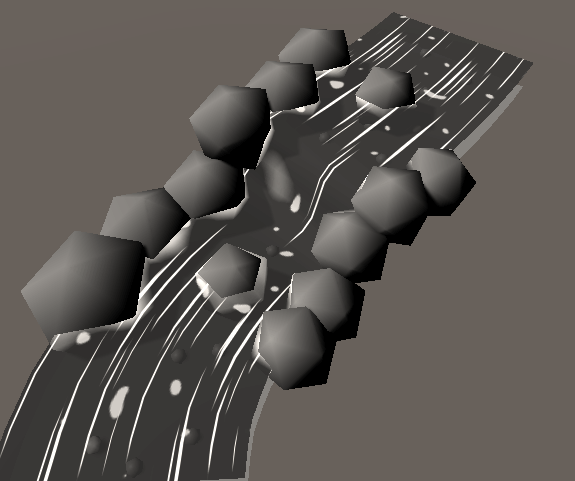We’ll start with a transparent surface shader, for that we’ll have to add the `alpha` attribute to our surface shader declaration. Then we’ll change the tags so the rendertype is `"Transparent"`, it uses the `"Transparent"` Queue index and we’ll disable shadows for the material by setting `"ForceNoShadowCasting"` to `"True"`. Last we set the shader target to 4.0, that will allow us to use more interpolators(variables in the vertex to fragment struct) later that we’ll need.

``````//in subshader

//in CGPROGRAM
#pragma surface surf Standard fullforwardshadows alpha
#pragma target 4.0
``````

Then we’ll just render the base color of the river. We’ll set up a property for it. Then in the surface function we’ll apply the value to a color variable and write the rgb values to the albedo variable of the output struct and the alpha to the alpha variable of the output struct. We won’t touch the metallic or smoothness values, simply because changing them won’t fit the style I’m trying to archieve, but I encourage you to change them and see how result looks with different values.

``````Properties {
_Color ("Base Color", Color) = (1,1,1,1)
}
``````
``````//shader variables
fixed4 _Color;
``````
``````void surf (Input IN, inout SurfaceOutputStandard o) {
float4 col = _Color;
o.Albedo = col.rgb;
o.Alpha = col.a;
}
``````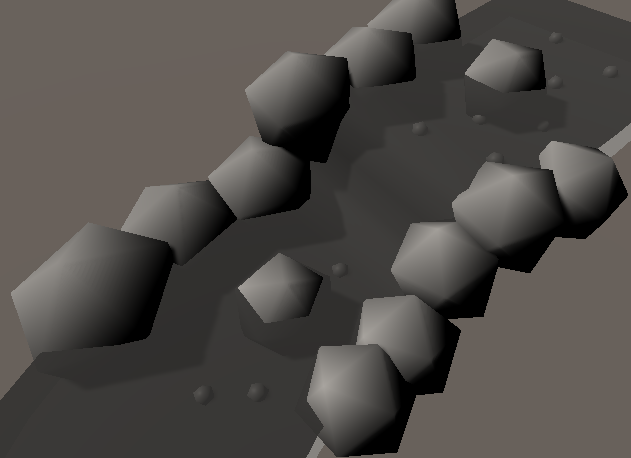## Scrolling UVs

Next we’ll add a scrolling texture to the shader. First we’ll add the properties we need. The texture itself, the tint of the texture, and the direction it scrolls in. Above those variables we add a header to show to the user that those variables are to regulate the first layer of specs. For the uv coordinates of the texture we’ll add a variable that’s called `uv`+`TextureName` to our input struct.

``````Properties {
_Color ("Base Color", Color) = (1,1,1,1)

_Specs1 ("Specs", 2D) = "white" {}
_SpecColor1 ("Spec Color", Color) = (1,1,1,1)
_SpecDirection1 ("Spec Direction", Vector) = (0, 1, 0, 0)
}
``````
``````struct Input {
float2 uv_Specs1;
}
``````
``````//shader variables
sampler2D _Specs1;
fixed4 _SpecColor1;
float2 _SpecDirection1;
``````

Then after we set that up, we can use the scrolling in the surface shader. First we calculate the spec coordinates by adding the direction of the scrolling multiplied by the time to the base coordinates of the texture. We get the time by accessing the `_Time.y` variable. In the y parameter is the unscaled time while the x, z, and w paramters store the time scaled by different factors.

Then we access the specs texture at the calculated coordinates and multiply it by the tint. After we did that we can use mix it with the existing color. First we interpolate from the rgb components of the color so far to the rgb components of the specs based on the alpha channel of the specs and apply it to the rgb components of the color. Then we interpolate from the alpha value of the color so far to 1 based on the alpha channel of the spec color and apply the result to the alpha channel of our color. By interpolating from the base color to the new color based on the alpha value we make it so when the new texture has a alpha value of 0 (is completely transparent), it doesn’t change the color at all. If it’s completely visible(alpha of 1), we use the new color. The reason that we do the alpha value separately is that if we would interpolate the whole color based on the alpha of the spec color, it could lower the alpha value at some parts, making the surface more see-through, but we always want add to the alpha, so interpolating from the existing value to being completely opaque gives us the results we want.

``````void surf (Input IN, inout SurfaceOutputStandard o) {
fixed4 col = _Color;

float2 specCoordinates1 = IN.uv_Specs1 + _SpecDirection1 * _Time.y;
fixed4 specLayer1 = tex2D(_Specs1, specCoordinates1) * _SpecColor1;
col.rgb = lerp(col.rgb, specLayer1.rgb, specLayer1.a);
col.a = lerp(col.a, 1, specLayer1.a);

/*fixed4 specLayer2 = tex2D(_Specs2, IN.uv_Specs2 + _SpecDirection2 * _Time.y);
col.rgb = lerp(col.rgb, specLayer2.rgb * _SpecColor2, specLayer2.a);
col.a = col.a + specLayer2.a;

float4 projCoords = UNITY_PROJ_COORD(IN.screenPos);
float sceneZ = LinearEyeDepth(rawZ);
float partZ = IN.eyeDepth;

float foam = 1-saturate((sceneZ - partZ) / _FoamAmount);
float foamNoise = tex2D(_FoamNoise, IN.uv_FoamNoise + _FoamDirection * _Time.y);
foam = saturate(foam - foamNoise);

col.rgb = lerp(col.rgb, _FoamColor, foam);
col.a += foam;*/

o.Albedo = col.rgb;
o.Alpha = col.a;
}
``````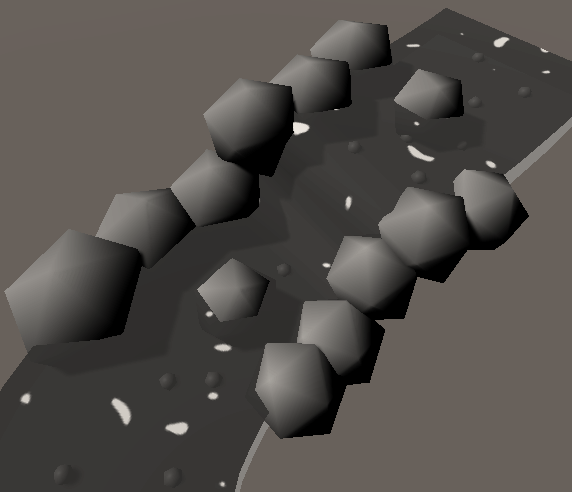For this to word it’s important to set up your river mesh the correct way! The coordinates have to be continous and along the flow of the river. I use blender for modelling and my unwrapping workflow is to autounwrap the whole river once automatically, then I choose a random quad and scale the right and left edge in the x direction to 0 so they’re completely vertical, then I scale the upper and lower edge in the y direction so they’re completely horizonal. After that I select the corrected quad and unwrap the whole river again with the “follow active quads” mode. You can make the river move faster in some places by changing it so the uv coordinates of the vertices are closer together at those places. (I did that where the water goes down a bit)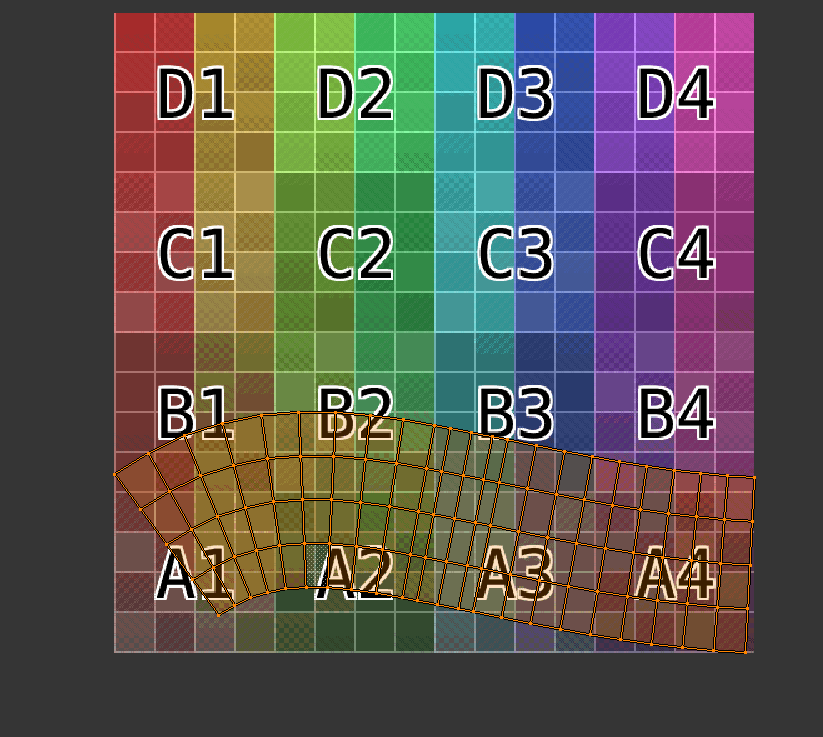For the specs texture, I did a cutoff on a perlin noise and baked that into a texture via my material baking tool. Here is the texture:And here are the other values I used: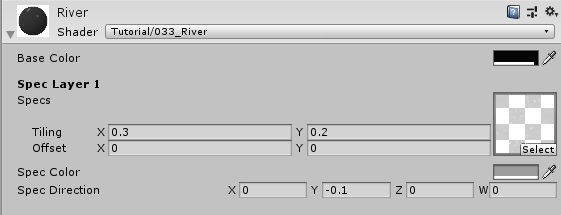## Multiple scrolling textures

We can simply repeat this process to add another scrolling texture to the river. This new texture will override the color of the old one where it’s visible. So we’ll add a new specs texture, tint and scrolling direction as well as a uv set in the input struct.

``````//in properties
_Specs2 ("Specs", 2D) = "white" {}
_SpecColor2 ("Spec Color", Color) = (1,1,1,1)
_SpecDirection2 ("Spec Direction", Vector) = (0, 1, 0, 0)
``````
``````//in shader variables
sampler2D _Specs2;
fixed4 _SpecColor2;
float2 _SpecDirection2;
``````
``````//input struct
struct Input {
float2 uv_Specs1;
float2 uv_Specs2;
}
``````
``````//in surface function
float2 specCoordinates2 = IN.uv_Specs2 + _SpecDirection2 * _Time.y;
fixed4 specLayer2 = tex2D(_Specs2, specCoordinates2) * _SpecColor2;
col.rgb = lerp(col.rgb, specLayer2.rgb, specLayer2.a);
col.a = lerp(col.a, 1, specLayer2.a);
``````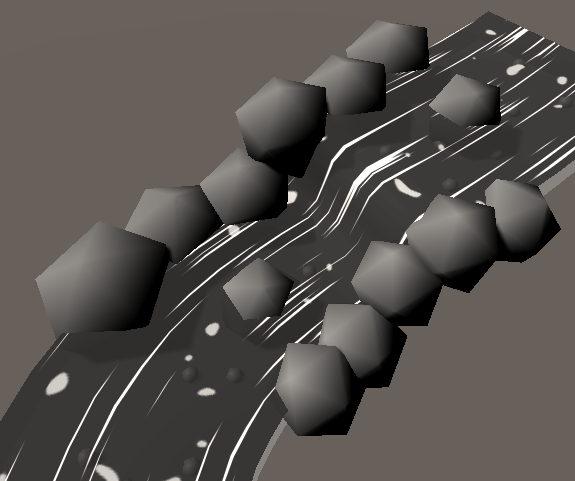To make the new specs texture I also used a cutoff perlin noise, but with a higher frequency and lower cutoff value. It’s this texture:And the other settings for the second specs layer look like this: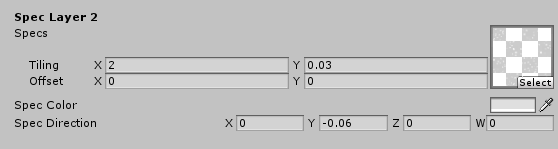## Foam

As a last detail to the shader we will add foam near objects in the water. For this we will use a very common technique where we’ll compare the depth of the object behind the water with the depth of the water surface.

Before we start, we set up the properties of the foam. We’ll use a noise texture to make the foam vary over time, it’ll also need a custom uv parameter in the input struct. Then we’ll use a direction to make the noise move, just like we did with the specs earlier. And we add a color for the foam and a variable to regulate how visible in the water the shader will check for objects.

``````//in properties
_FoamNoise("Foam Noise", 2D) = "white" {}
_FoamDirection ("Foam Direction", Vector) = (0, 1, 0, 0)
_FoamColor ("Foam Color", Color) = (1,1,1,1)
_FoamAmount ("Foam Amount", Range(0, 2)) = 1
``````
``````//in shader variables
sampler2D _FoamNoise;
fixed4 _FoamColor;
float _FoamAmount;
float2 _FoamDirection;
``````
``````//input struct
struct Input {
float2 uv_Specs1;
float2 uv_Specs2;
float2 uv_FoamNoise;
}
``````

We start by getting the depth of the surface. Because surface shaders don’t have a function that can generate them for us, we have to write our own vertex function. First we add it to our surface shader declaration, then we write it. In it we just initialize the input struct for the surface function, then we write the depth to it via the COMPUTE_EYEDEPTH macro. To save it into the input struct we’ll extend it to also hold a eyedepth variable.

``````//surface shader declaration
#pragma surface surf Standard vertex:vert fullforwardshadows alpha
``````
``````//input struct
struct Input {
float2 uv_Specs1;
float2 uv_Specs2;
float2 uv_FoamNoise;
float eyeDepth;
};
``````
``````void vert (inout appdata_full v, out Input o)
{
UNITY_INITIALIZE_OUTPUT(Input, o);
COMPUTE_EYEDEPTH(o.eyeDepth);
}
``````

Then we get the depth behind the surface by reading from the depth texture. So we add a new texture variable called _CameraDepthTexture. By adding it to our shader it will be automatically assigned. To read from it we have to get the screen coordinates, luckily we can get them easily by adding a variable called `screenPos` to our input struct.

In the surface function we can then get the projection coordinate by passing the screenPos to the `UNITY_PROJ_COORD` macro. With it we can sample the depth texture by passing the depth texture as well as the projection coordinates to the `SAMPLE_DEPTH_TEXTURE_PROJ` macro to get the raw depth. The last step to get the scene depth from that is to simply pass it to the `LinearEyeDepth` function.

``````//input struct
struct Input {
float2 uv_Specs1;
float2 uv_Specs2;
float2 uv_FoamNoise;
float eyeDepth;
float4 screenPos;
}
``````
``````//shader variables
``````
``````//in surface function
float4 projCoords = UNITY_PROJ_COORD(IN.screenPos);
float sceneZ = LinearEyeDepth(rawZ);
float surfaceZ = IN.eyeDepth;
``````

Now that we have this data we can compare it. We simply subtract the depth of the surface from the depth of the scene. Then we divide it by the amount of foam, so the more foam we have, the slower the value grows. This value will start at 0 when the surface below is very close and grow the further away it is. Instead we want the foam to start at full opacity and become less visible the further away the ground gets, so we subtract it from one. Then as a last step before rendering the foam we’ll clamp it between 0 and 1 to avoid weird interpolation artefacts.

``````float foam = 1-((sceneZ - surfaceZ) / _FoamAmount);
foam = saturate(foam);

o.Albedo = foam;
o.Alpha = 1;
return;
``````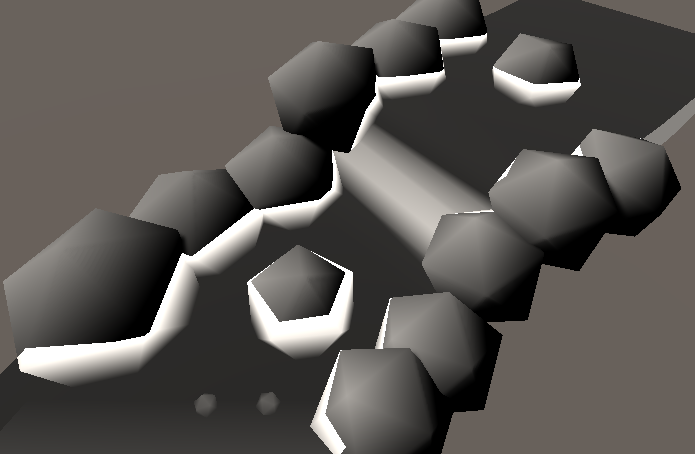Then that we have the foam we can mix it into the color just the we did with the specs. Because the foam is just a simple value and not a color, we multiply it with the foam color alpha manually, this allows us to make the foam factor in less by lessening the alpha of it’s color.

``````float foam = 1-((sceneZ - surfaceZ) / _FoamAmount);
foam = saturate(foam);
col.rgb = lerp(col.rgb, _FoamColor.rgb, foam);
col.a = lerp(col.a, 1, foam * _FoamColor.a);
``````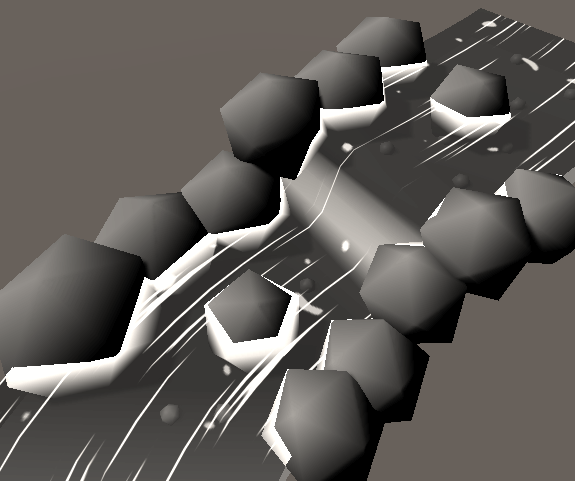To make the foam more interresting and lively, we then subtract a noise texture from it. We calculate the uv coordinates for it just like before - by adding the direction multiplied by the time to the base coordinates. Then we read the texture, but only use the red channel because the foam is a simple value without color. The subtraction of of the texture from the foam is just before the clamping.

``````float2 foamCoords = IN.uv_FoamNoise + _FoamDirection * _Time.y;
float foamNoise = tex2D(_FoamNoise, foamCoords).r;
float foam = 1-((sceneZ - surfaceZ) / _FoamAmount);
foam = saturate(foam - foamNoise);
col.rgb = lerp(col.rgb, _FoamColor.rgb, foam);
col.a = lerp(col.a, 1, foam * _FoamColor.a);
``````

With this we have a complete river shader.For the foam noise I used a normal tiling perlin noise baked into a texture. This is the texture: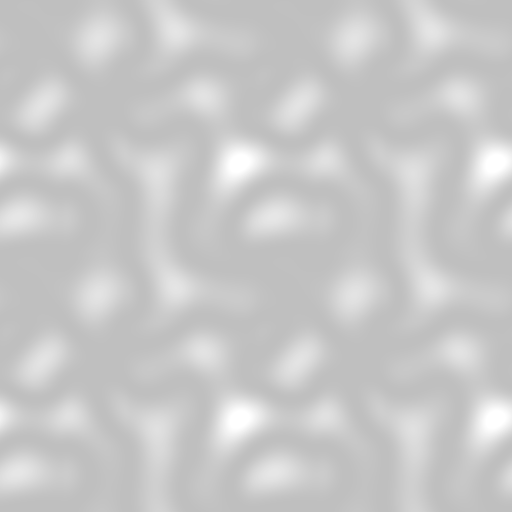And those are all settings I used for the material: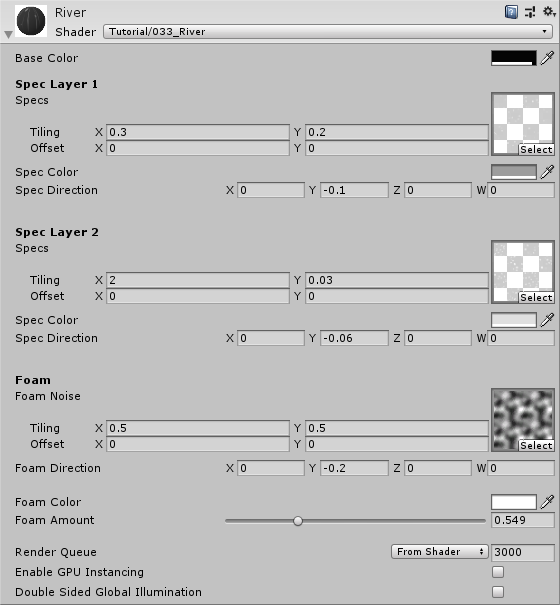## Source

``````Shader "Tutorial/033_River" {
Properties {
_Color ("Base Color", Color) = (1,1,1,1)

_Specs1 ("Specs", 2D) = "white" {}
_SpecColor1 ("Spec Color", Color) = (1,1,1,1)
_SpecDirection1 ("Spec Direction", Vector) = (0, 1, 0, 0)

_Specs2 ("Specs", 2D) = "white" {}
_SpecColor2 ("Spec Color", Color) = (1,1,1,1)
_SpecDirection2 ("Spec Direction", Vector) = (0, 1, 0, 0)

_FoamNoise("Foam Noise", 2D) = "white" {}
_FoamDirection ("Foam Direction", Vector) = (0, 1, 0, 0)
_FoamColor ("Foam Color", Color) = (1,1,1,1)
_FoamAmount ("Foam Amount", Range(0, 2)) = 1
}
LOD 200

CGPROGRAM
// Physically based Standard lighting model, and enable shadows on all light types, then set it to render transparent
#pragma surface surf Standard vertex:vert fullforwardshadows alpha

#pragma target 4.0

struct Input {
float2 uv_Specs1;
float2 uv_Specs2;
float2 uv_FoamNoise;
float eyeDepth;
float4 screenPos;
};

fixed4 _Color;

sampler2D _Specs1;
fixed4 _SpecColor1;
float2 _SpecDirection1;

sampler2D _Specs2;
fixed4 _SpecColor2;
float2 _SpecDirection2;

sampler2D _FoamNoise;
fixed4 _FoamColor;
float _FoamAmount;
float2 _FoamDirection;

void vert (inout appdata_full v, out Input o)
{
UNITY_INITIALIZE_OUTPUT(Input, o);
COMPUTE_EYEDEPTH(o.eyeDepth);
}

void surf (Input IN, inout SurfaceOutputStandard o) {
//set river base color
fixed4 col = _Color;

//add first layer of moving specs
float2 specCoordinates1 = IN.uv_Specs1 + _SpecDirection1 * _Time.y;
fixed4 specLayer1 = tex2D(_Specs1, specCoordinates1) * _SpecColor1;
col.rgb = lerp(col.rgb, specLayer1.rgb, specLayer1.a);
col.a = lerp(col.a, 1, specLayer1.a);

//add second layer of moving specs
float2 specCoordinates2 = IN.uv_Specs2 + _SpecDirection2 * _Time.y;
fixed4 specLayer2 = tex2D(_Specs2, specCoordinates2) * _SpecColor2;
col.rgb = lerp(col.rgb, specLayer2.rgb, specLayer2.a);
col.a = lerp(col.a, 1, specLayer2.a);

//get scene and surface depth
float4 projCoords = UNITY_PROJ_COORD(IN.screenPos);
float sceneZ = LinearEyeDepth(rawZ);
float surfaceZ = IN.eyeDepth;

float2 foamCoords = IN.uv_FoamNoise + _FoamDirection * _Time.y;
float foamNoise = tex2D(_FoamNoise, foamCoords).r;
float foam = 1-((sceneZ - surfaceZ) / _FoamAmount);
foam = saturate(foam - foamNoise);
col.rgb = lerp(col.rgb, _FoamColor.rgb, foam);
col.a = lerp(col.a, 1, foam * _FoamColor.a);

//apply values to output struct
o.Albedo = col.rgb;
o.Alpha = col.a;
}
ENDCG
}
FallBack "Diffuse"
}
``````

I hope this tutorial helped you to create a nice looking river. If you have any questions about existing tutorials or requests for new ones, just write me.

You can also find me on twitter at @totallyRonja. If you liked my tutorial and want to support me you can do that on Patreon (patreon.com/RonjaTutorials) or Ko-Fi (ko-fi.com/RonjaTutorials).